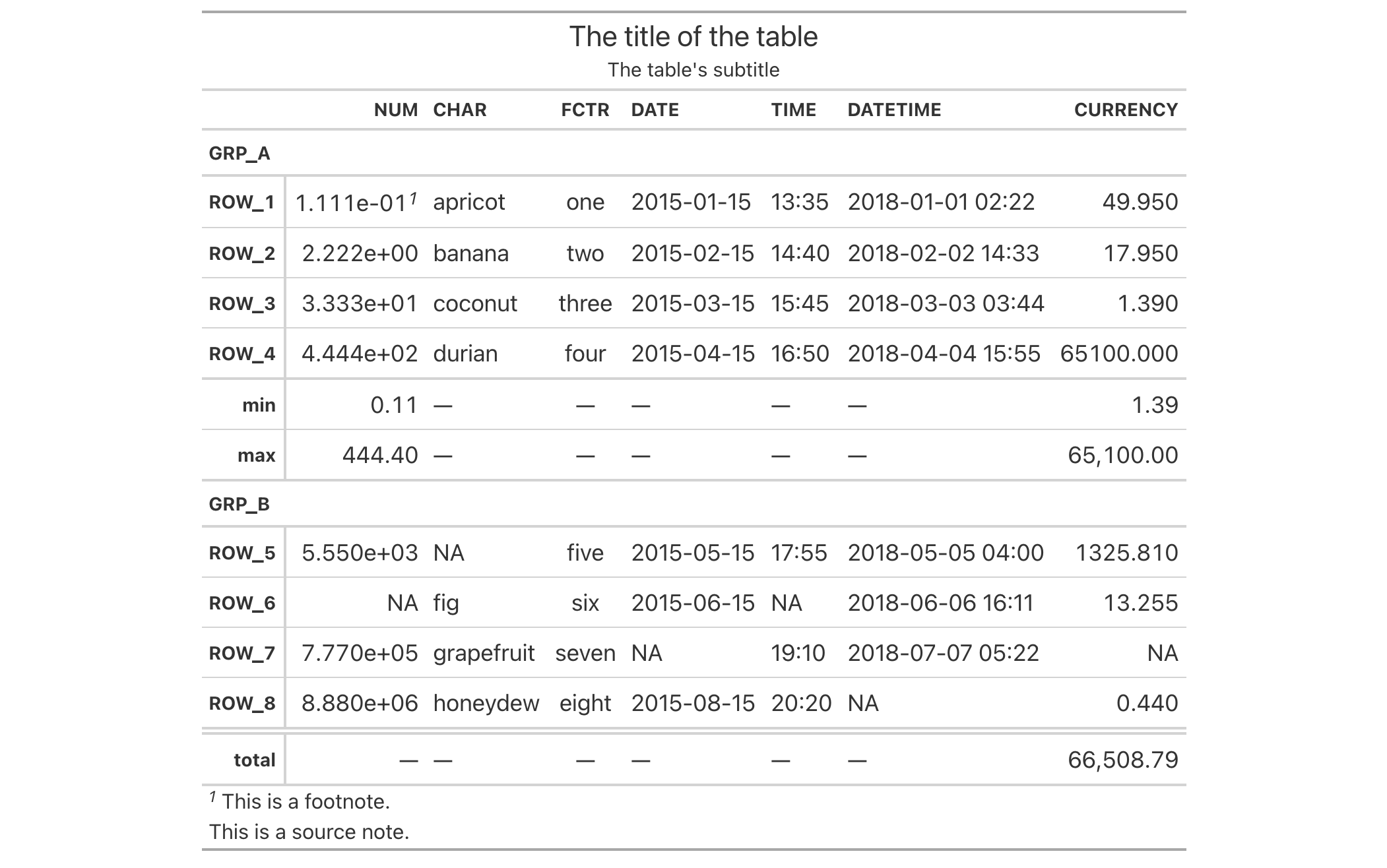# opt_all_caps: Option to use all caps in select table locations In gt: Easily Create Presentation-Ready Display Tables

 opt_all_caps R Documentation

## Option to use all caps in select table locations

### Description

Sometimes an all-capitalized look is suitable for a table. With the `opt_all_caps()` function, we can transform characters in the column labels, the stub, and in all row groups in this way (and there's control over which of these locations are transformed).

This function serves as a convenient shortcut for `<gt_tbl> %>% tab_options(<location>.text_transform = "uppercase", <location>.font.size = pct(80), <location>.font.weight = "bolder")` (for all `locations` selected).

### Usage

```opt_all_caps(
data,
all_caps = TRUE,
locations = c("column_labels", "stub", "row_group")
)
```

### Arguments

 `data` A table object that is created using the `gt()` function. `all_caps` A logical value to indicate whether the text transformation to all caps should be performed (`TRUE`, the default) or reset to default values (`FALSE`) for the `locations` targeted. `locations` Which locations should undergo this text transformation? By default it includes all of the `"column_labels"`, the `"stub"`, and the `"row_group"` locations. However, we could just choose one or two of those.

### Value

An object of class `gt_tbl`.

### Examples

Use `exibble` to create a gt table with a number of table parts added. All text in the column labels, the stub, and in all row groups is to be transformed to all caps using `opt_all_caps()`.

```exibble %>%
gt(rowname_col = "row", groupname_col = "group") %>%
summary_rows(
groups = "grp_a",
columns = c(num, currency),
fns = list(
min = ~min(., na.rm = TRUE),
max = ~max(., na.rm = TRUE)
)) %>%
grand_summary_rows(
columns = currency,
fns = list(
total = ~sum(., na.rm = TRUE)
)) %>%
tab_source_note(source_note = "This is a source note.") %>%
tab_footnote(
footnote = "This is a footnote.",
locations = cells_body(columns = 1, rows = 1)
) %>%
title = "The title of the table",
subtitle = "The table's subtitle"
) %>%
opt_all_caps()
```### Function ID

9-6

Other table option functions: `opt_align_table_header()`, `opt_css()`, `opt_footnote_marks()`, `opt_horizontal_padding()`, `opt_row_striping()`, `opt_stylize()`, `opt_table_font()`, `opt_table_lines()`, `opt_table_outline()`, `opt_vertical_padding()`